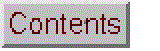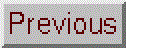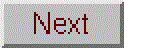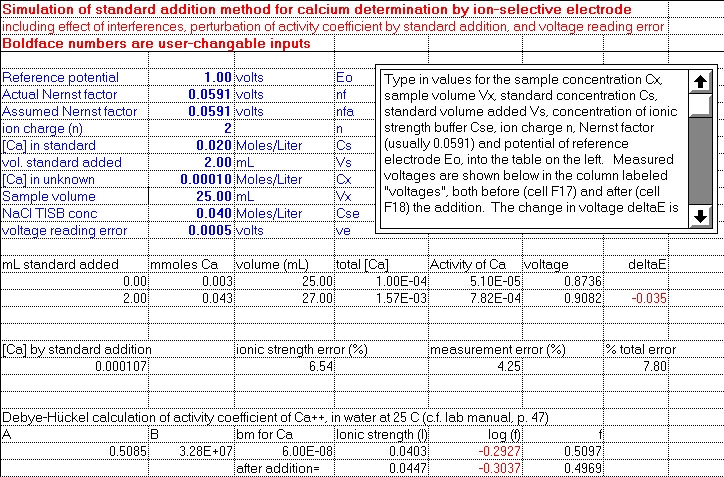# Calcium Ion Selective Electrode SimulationScreen shot. Click to see larger graphic.

### [Operating instructions] [Cell definitions and equations] [Student assignment handout]

Simulation of the standard addition method for calcium determination by ion-selective electrode. The simulation demonstrates the ability of the standard addition method to correct for an unknown reference potential and unknown  ionic strength of the solution (and thus activity coefficient). It includes two sources of error: the effect of the addition of standard on the ionic strength and activity of calcium, and effect of voltage reading error. Further reading:  A Beginners Guide to Ion-Selective Electrode Measurements, by  Chris C. Rundle.

WingZ format: CaElectrode.wkz
Wingz player application and basic set of simulation modules, for windows PCs or Macintosh

OpenOffice Calc format: CaElectrode.ods
Excel format: CaElectrode.xls
View equations in PDF format

Other related simulations:
Error propagation in Analytical Calibration (single standard additon)

Operating instructions:
Type in values for the sample concentration Cx, sample volume Vx, standard concentration Cs, standard volume added Vs, concentration of ionic strength buffer Cse, ion charge n, Nernst factor (usually 0.0591) and potential of reference electrode Eo, into the table on the left. Measured voltages are shown below in the column labeled "voltages", both before (cell F17) and after (cell F18) the addition. The change in voltage deltaE is shown in cell G18, and the concentration calculated from the standard addition equation is shown in cell A22 (Click on this cell to display the equation in the entry bar at the top of the window). Compare the calculated value in cell A22 to the "correct" value Cx in cell B11. Note that the reference potential (Eo) has an effect on the measured voltages but no effect on deltaE nor on the calculated concentration. This calculated concentration won't be perfect, however, because adding the standard solution to the sample causes a slight increase in its ionic strength and thus a slight decrease in the activity coefficient of calcium. The percent difference between the calculated and true values of Cx is given in cell C22. You can reduce this error by using a smaller addition of standard (reduce Cs or Vx or both) or by using a greater concentration of NaCl ionic strength buffer (Cse, in cell B13). Using too much NaCl, however, will increase the interference of Na ions (c.f. the table of interference factors in rows 39-49). On the other hand, reducing the amount of standard added reduces deltaE and makes it harder to measure precisely. This effect can be simulated by specifying a voltage reading error in cell B14. The resulting % error in concentration is shown in cell E22, and the total error is shown in cell G22.

Cell definitions and equations:
```Inputs:

Reference potential   (volts)       Eo  (cell B5)
Actual Nernst factor  (volts)       nf  (cell B6)
Assumed Nernst factor (volts)       nfa (cell B7)
ion charge (n)        (unitless)    n   (cell B8)
[Ca] in standard      (Moles/Liter) Cs  (cell B9)
vol. standard added   (mL)          Vs  (cell B10)
[Ca] in unknown	      (Moles/Liter) Cx  (cell B11)
Sample volume         (mL)          Vx  (cell B12)
NaCl TISB conc        (Moles/Liter) Cse (cell B13)
voltage reading error (volts)       ve  (cell B14)

Debye-Hückel factors:
A = 0.5085 (cell A26)
B = 3.28E+07 (cell A27)
bm = 6.00E-08 (cell A28)

Calculated quantities:

mmoles Ca   volume   total [Ca]   Activity of Ca   measured voltage
mm1=Cx*Vx   V1=Vx    Ca1=mm1/V1   aCa1=f1*Ca1      V1=Eo+(nf/n)*log(aCa1+totalAE)

After addition of Vs ml of standard solution
mmoles Ca       volume    total [Ca]  Activity of Ca measured voltage
mm2=Cx*Vx+Cs*Vs V2=Vx+Vs  Ca2=mm2/V2  aCa2=f2*Ca2   V2=Eo+(nf/n)*log(aCa2+totalAE)

deltaE = V2-V1

Casa = Cs*Vs/((Vx+Vs)*10^(-n*deltaE/nfa)-Vx)

ionic strength error (%)
ise = 100*(Casa-Cx)/Cx

measurement error (%)
ma = 100*((Cs*Vs/((Vx+Vs)*10^(-n*(deltaE+ve)/nfa)-Vx))-Casa)/Casa

% total error
=sqrt(ise*ise+ma*ma)

Debye-Hückel calculation of activity coefficient of Ca+2, in water at 25 C:

Ionic strength   log activity coefficient          activity coefficient

Effect of interferences from ions in commercial NaCl ionic strength buffer solutionIon   Atomic   selectivity    ion      µg/mL in        M in     activity    activity       weight    constant*    charge    solution**    solution  in solution  equivalence---------------------------------------------------------------------------------------H+    1.008    10000000         1    (assume pH=7)  1.0E-07       5.1E-08     2.6E-08Cu++  63.55      0.3            2        0.02       9.7E-08       4.9E-08     1.5E-08Mg++  24.30      0.01           2       20          2.3E-04       1.2E-04     1.2E-06Na+   22.99      0.0016         1      100          =Cse          =f1*Cse     6.9E-07                                                                              -------* from the electrode's spec sheet                                   totalAE = 1.9E-06** from the reagent label
```
Student Activity Handout:

CaElectrode.wkz is a spreadsheet simulation of the
standard addition method for calcium determination by ion-selective electrode. The simulation demonstrates the ability of the standard addition method to correct for an unknown reference potential and ionic strength (and thus activity coefficient). It includes two sources of error: (1) the effect of the addition of standard on the ionic strength and (2) effect of voltage reading error.

Looking at the screen display, on the top right is a scrolling text field that contains a summary of these instructions. On the top left is a table of all of the model parameters that you can control. These include the potential of reference electrode Eo, the Nernst factor (with separate values for the "actual" Nernst factor used to calculate the voltages and the "assumed" Nernst factor used in the standard addition equation), ion charge n, concentration of calcium in the standard Cs, standard volume added Vs, concentration of calcium in the "unknown" sample Cx, sample volume Vx, concentration of the NaCl ionic strength buffer Cse, and the voltage reading error, i.e. the precision with which voltages can be read. The current values of these parameters are shown in boldface type in column B; you can change any of these parameters simply by clicking on the number, typing a new value, and pressing the enter key.

The bottom half of the screen shows all the calculated "outputs" of the simulation. Rows 17 and 18 show the status of the sample solution before and after the addition of standard, respectively. Measured voltages are shown below in the column labeled "voltages", both before the addition of standard (cell F17) and after the addition (cell F18). The change in voltage delta-E is shown in cell G18, and the concentration calculated from the standard addition equation is shown in cell A22. (Click on this cell to display the equation in the entry bar at the top of the window; compare to the equation on page 48 of the lab manual).

1. Compare the calculated value in cell A22 to the "correct" value Cx in cell B11. Change the reference potential (Eo) and note the effect it has on the measured voltages and on delta-E. Does the reference have an effect on the calculated calcium concentration?

2. Change the actual and assumed Nernst factors, keeping them equal. Does this effect the measured voltages? Does this effect the delta-E? Does this effect the calculated calcium concentration? What if you make the actual and assumed Nernst factors unequal? Will the standard addition method give accurate results if the Nernst factor of the electrode is not known?

3. Note that adding standard solution to the sample causes a slight increase in its ionic strength and thus a slight difference in the activity coefficient of calcium. Compare cells D27 and D28, which show the ionic strength of the sample before and after the addition, respectively. The resulting effect on the activity coefficient for calcium can be calculated from the Debye-Huckel equation (Wikipedia; lab manual, p. 47) and the results are shown in cells F27 and F28. The change in activity coefficient gives rise to an error in the determination, because the electrode potential responds to activity, not concentration. The percent difference between the calculated and true values of Cx is given in cell C22. You can reduce this error by using a smaller addition of standard (reduce Cs or Vx or both) or by using a greater concentration of ionic strength buffer (Cse, in cell B13). Try it.

4. Reducing the concentration and/or volume of the added standard reduces the error due to ionic strength changes, but it also reduces deltaE and makes it harder to measure precisely. This effect can be simulated by specifying a voltage reading error ( i.e. the precision with which voltages can be read) in cell B14. The resulting % error in concentration is shown in cell E22, and the total error (quadratic sum of voltage error and ionic strength error) is shown in cell G22. Set the voltage reading error to 0.0001 volts (the least significant digit of the pH meters we use) and determine if there is a value of Vs that gives a minimum overall error. In practice, a more realistic value for the voltage reading error is 0.0005 volts. With that value, what dominates the total error?

(c) 1991, 2015. This page is part of Interactive Computer Models for Analytical Chemistry Instruction, created and maintained by Prof. Tom O'Haver , Professor Emeritus, The University of Maryland at College Park. Comments, suggestions and questions should be directed to Prof. O'Haver at toh@umd.edu. Number of unique visits since May 17, 2008: